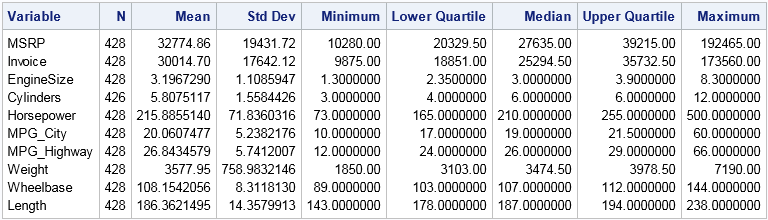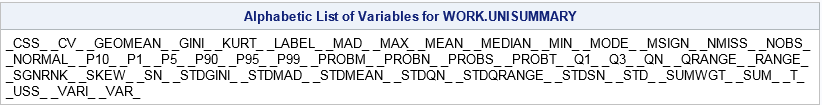Descriptive univariate statistics are the foundation of data analysis. Before you create a statistical model for new data, you should examine descriptive univariate statistics such as the mean, standard deviation, quantiles, and the number of nonmissing observations.

In SAS, there is an easy way to create a data set that contains the descriptive statistics for every numerical variable in your data: use the OUTTABLE= option in PROC UNIVARIATE. It doesn't matter if your data has 5 variables or 5,000 variables. That one option writes dozens of statistics for all numerical variables in the data!

### PROC MEANS: A great way to display statistics on the screen

First, some basic background about viewing descriptive statistics. Many people learned that PROC MEANS is the standard way to display descriptive statistics on your computer screen. You specify the statistics that you want on the PROC MEANS statement, as follows:

```/* can produce 38 descriptive statistics */ proc means data=sashelp.cars nolabels N Mean Std Min Q1 Median Q3 Max; /* specify the statistics */ run; /* omit VAR statement to analyze all numerical variables */```Notice that by omitting the VAR statement, the procedure analyzes all numerical variables. The output is an ODS table named "Summary."

That was easy. However, suppose that you do not care about seeing the statistics on your computer monitor, but you want them written to a SAS data set. Because you are analyzing many variables and want many statistics, it is somewhat awkward to use the OUTPUT statement in PROC MEANS. An easier way is to use ODS to write the statistics to a data set by doing the following:

• Use the ODS EXCLUDE statement to suppress output to the monitor.
• Specify the statistics that you want to see: the mean, standard deviation, median, and so forth.
• Specify the STACKODSOUTPUT option on the PROC MEANS statement to tell the procedure to preserve the tabular form of the statistics table.
• Use the ODS OUTPUT statement to write the "Summary" table to a SAS data set.

The solution now requires several lines of typing. It's not onerous, but it is a lot to remember:

```ods exclude all; /* suppress display to open ODS destinations */ proc means data=sashelp.cars N Mean Std Min Q1 MEDIAN Q3 Max /* type every statistic you want */ STACKODSOUTPUT; /* preserve table form of output */ ods output Summary=MeansSummary; /* write statistics to data set */ run; ods exclude none; /* restore normal display of tables */```

The data set MeansSummary contains the statistics for every numerical variable in the original data. This solution requires using ODS statements to direct the output to a data set while suppressing it from appearing on the screen. It is a nice trick in general, but there is an easier way for this particular task.

### PROC UNIVARIATE and the OUTTABLE= option

I've been using SAS for 20 years, but somehow I never learned about the OUTTABLE= option in PROC UNIVARIATE until last week. While reading the documentation I learned that the OUTTABLE= option creates an output data set that contains 46 descriptive statistics for each numerical variable on the VAR statement.

Cool #SAS option: easily write all descriptive #statistics to a data set
Click To Tweet

If you omit the VAR statement, PROC UNIVARIATE analyzes all numerical variables. Therefore, by specifying the OUTTABLE= option, you can duplicate the PROC MEANS example without using ODS. As an extra bonus, you do not have to type the names of any statistics.

The following statement computes 46 statistics for each numerical variable in the input data set. The data set UniSummary contains the results. The NORMAL option tells PROC UNIVARIATE to include the Kolmogorov-Smirnov test for normality in the statistics that it creates:

```proc univariate data=sashelp.cars outtable=UniSummary NORMAL noprint; run; /* omit VAR statement to analyze all numerical variables */   proc contents data=UniSummary short; run;```By default, PROC UNIVARIATE produces about two pages of tables for each numerical variable, but the NOPRINT option suppresses the display of tables. The output from PROC CONTENTS shows the names of variables in the output data set. Each name represents a statistic, such as CSS (cumulative sum of squares), CV (coefficient of variation), and GEOMEAN (geometric mean). The names begin and end with an underscore. The following statements print the same summary statistics that PROC MEANS produced; the output is not shown.

```proc print data=UniSummary label; var _var_ _label_ _NObs_ _Mean_ _Std_ _Min_ _Max_; run;```

For both procedures, you can use the CLASS statement to obtain statistics for subgroups of the data. For example, you can include the statement CLASS origin to obtain summary statistics for each variable and grouped according to whether a vehicle was manufactured in Europe, Asia, or the USA.

It is worth noting that PROC UNIVARIATE has options (for example, WINSORIZED= and TRIMMED=) that compute statistics that are not saved to the OUTTABLE= data set. However, you can use ODS to capture those statistics.

In summary, this article shows how to create a data set for descriptive statistics by using PROC MEANS or PROC UNIVARIATE. You can use either procedure, but using the OUTTABLE= option in PROC UNIVARIATE saves you some typing. The OUTTABLE= data set also includes some robust statistics that are not available in PROC MEANS.

The post Save descriptive statistics for multiple variables in a SAS data set appeared first on The DO Loop.